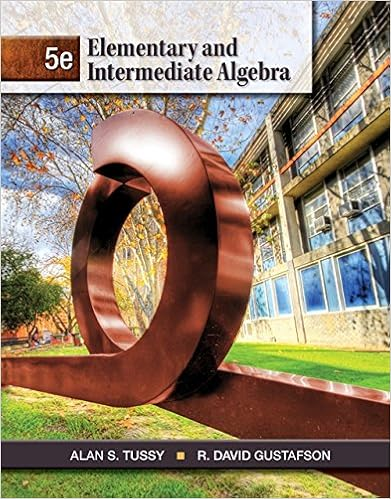# Chapter 16.rtf - Name Date A B C D A B C D A B C D 1.A...

• 9
• 96% (23) 22 out of 23 people found this document helpful

This preview shows page 1 - 3 out of 9 pages.

##### We have textbook solutions for you!
The document you are viewing contains questions related to this textbook.The document you are viewing contains questions related to this textbook.
Chapter 9 / Exercise 6
Elementary and Intermediate Algebra
Tussy/GustafsonExpert Verified
Name: __________________________ Date: _____________1.A level 0.95 confidence interval is:A)any interval with margin of error ± 0.95.B)a range of values computed from sample data that will contain the true value of the parameter of interest 95% of the time.C)a range of values with margin of error ± 0.95, which is also correct 95% of the time.D)a range of values computed from sample data by a method that guarantees that the probability the interval computed contains the parameter of interest is 0.95.
2.The upper 0.05 critical value of the standard Normal distribution is:
3.I collect a random sample of size n from a population and from the data collected compute a 95% confidence interval for the proportion I observe from the population. Which of the following would produce a new confidence interval with smaller width (smaller margin of error) based on these same data?
4.All confidence intervals have the form:
5.To calculate a 99% confidence interval, the value z* in the margin of error should equal:A)1.645.B)1.96.C)2.33.D)2.576.
6.Suppose the population standard deviation is = 5, an SRS of n= 100 is obtained, and Page 1
##### We have textbook solutions for you!
The document you are viewing contains questions related to this textbook.The document you are viewing contains questions related to this textbook.
Chapter 9 / Exercise 6
Elementary and Intermediate Algebra
Tussy/GustafsonExpert Verified
the confidence level is chosen to be 98%. The margin of error for estimating a mean is given by:
7.Suppose the population standard deviation is = 5, an SRS of n= 100 is obtained, and the confidence level is chosen to be 98%. The margin of error for estimating a mean is given by 1.1650. To reduce the margin of error to 0.3883 you should:
8.An SRS of size n0was taken to estimate mean body mass index (BMI) for girls between 13 and 19 years of age. The 95% confidence interval obtained had lower limit 19.5 and upper limit 26.3. Which statement is NOT true?
•••